The perfect place for easy learning...

×

# Java Selection Statements

In java, the selection statements are also known as decision making statements or branching statements or conditional control statements. The selection statements are used to select a part of the program to be executed based on a condition. Java provides the following selection statements.

• if statement
• if-else statement
• nested if statement
• if-else if statement
• switch statement

## if statement in java

In java, we use the if statement to test a condition and decide the execution of a block of statements based on that condition result. The if statement checks, the given condition then decides the execution of a block of statements. If the condition is True, then the block of statements is executed and if it is False, then the block of statements is ignored. The syntax and execution flow of if the statement is as follows.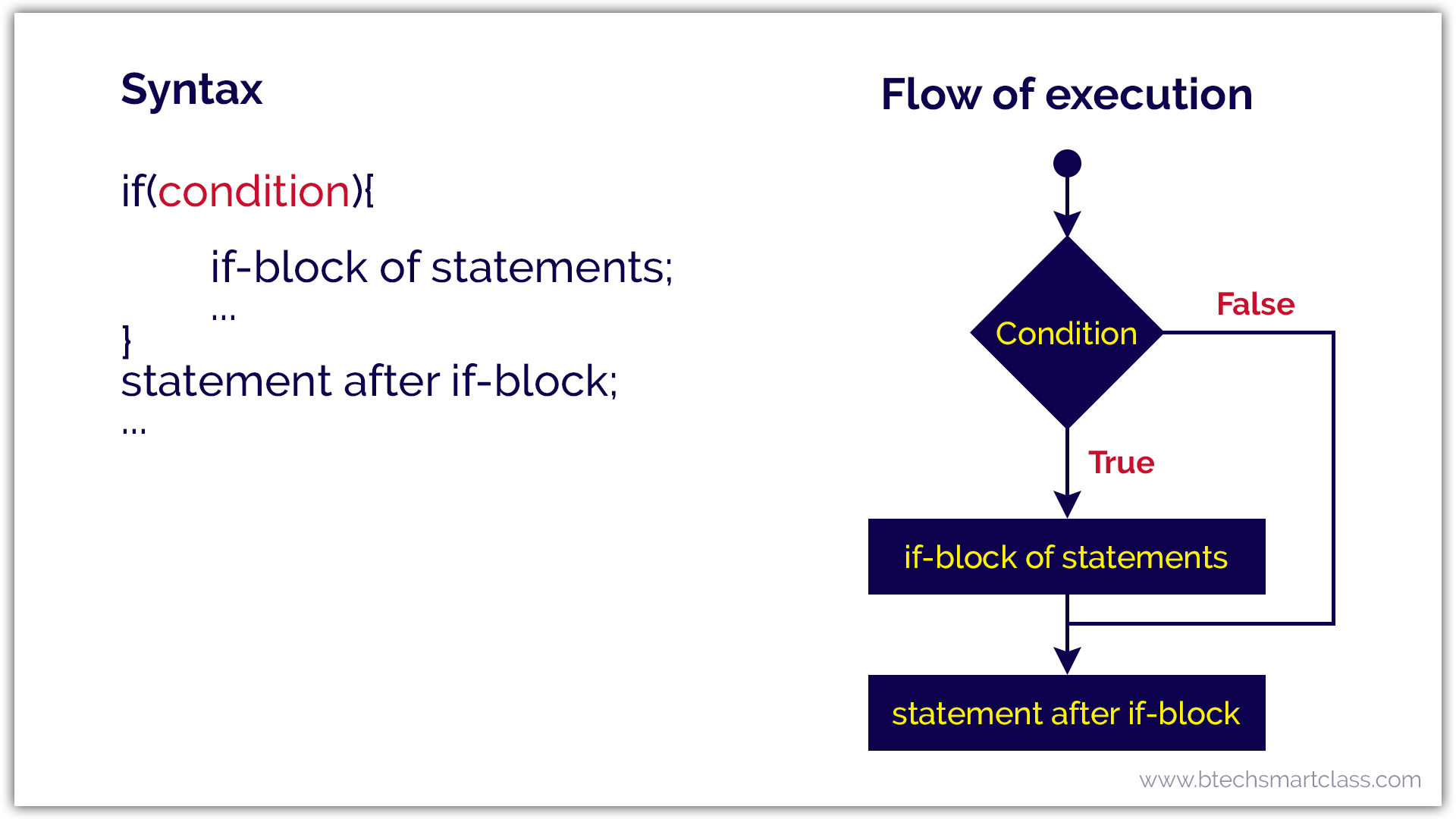Let's look at the following example java code.

Java Program
``````import java.util.Scanner;

public class IfStatementTest {

public static void main(String[] args) {

System.out.print("Enter any number: ");

if((num % 5) == 0) {
System.out.println("We are inside the if-block!");
System.out.println("Given number is divisible by 5!!");
}

System.out.println("We are outside the if-block!!!");

}

}```
```

When we run this code, it produce the following output.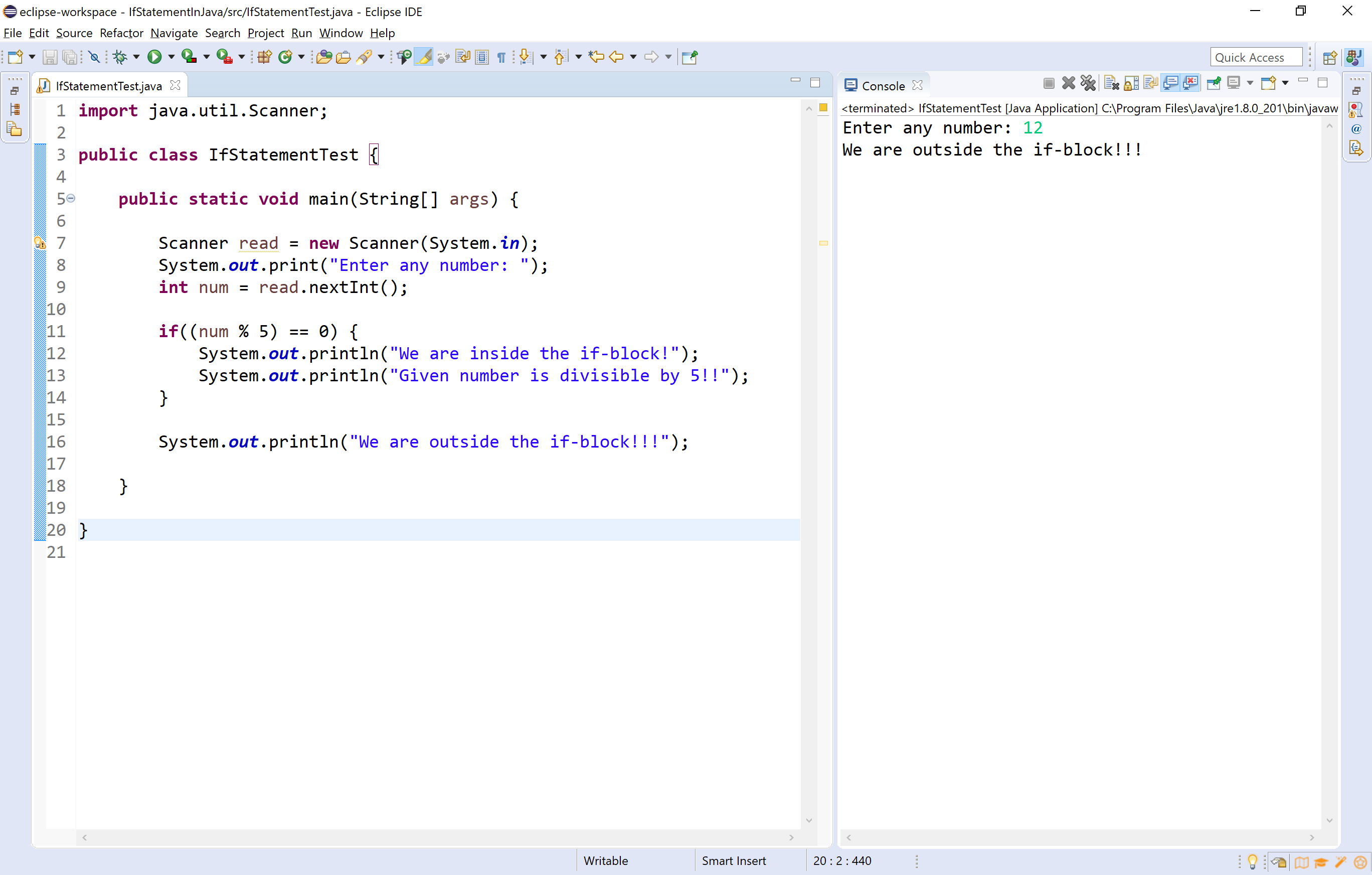In the above execution, the number 12 is not divisible by 5. So, the condition becomes False and the condition is evaluated to False. Then the if statement ignores the execution of its block of statements.

When we enter a number which is divisible by 5, then it produces the output as follows.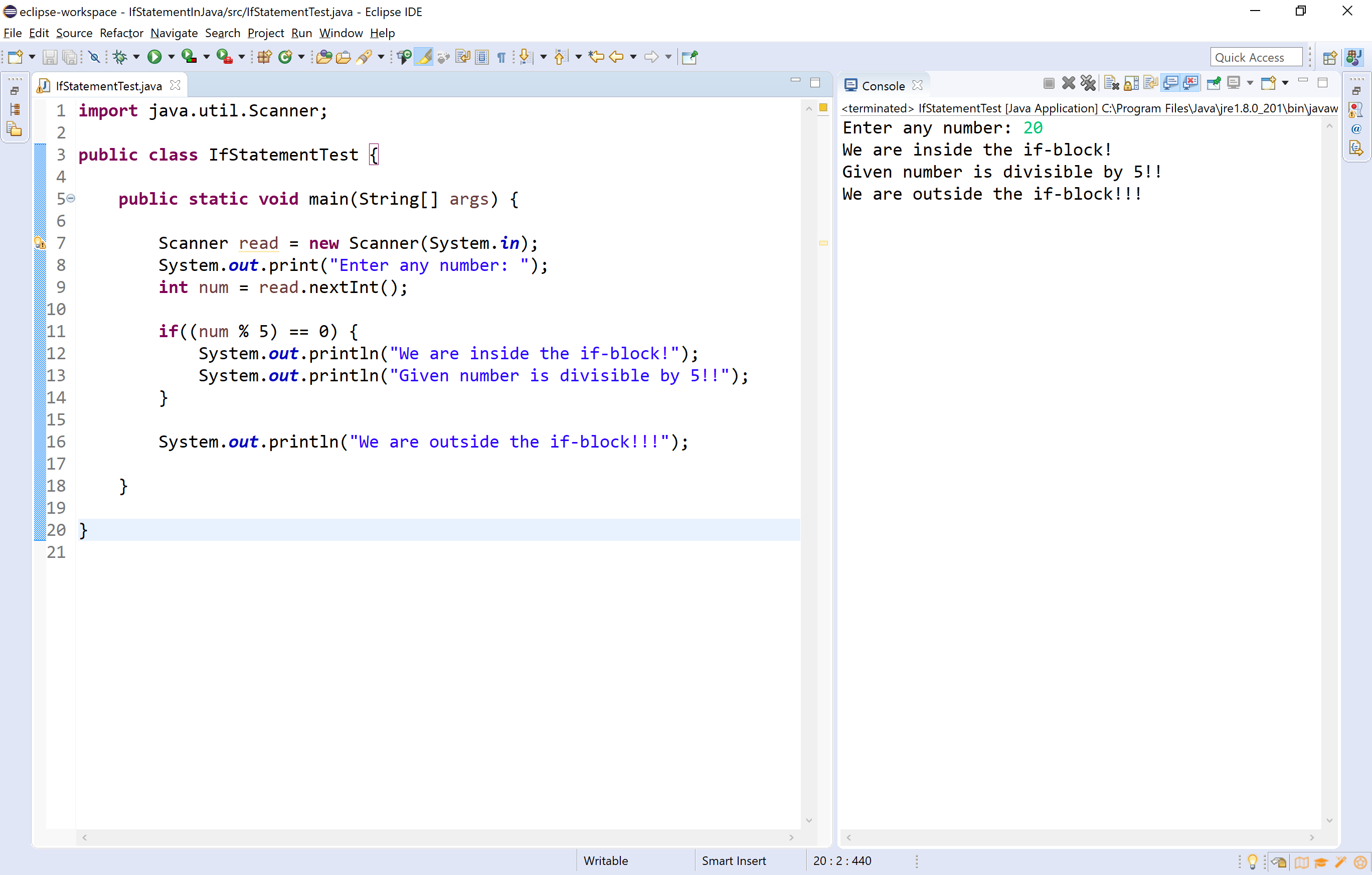## if-else statement in java

In java, we use the if-else statement to test a condition and pick the execution of a block of statements out of two blocks based on that condition result. The if-else statement checks the given condition then decides which block of statements to be executed based on the condition result. If the condition is True, then the true block of statements is executed and if it is False, then the false block of statements is executed. The syntax and execution flow of if-else statement is as follows.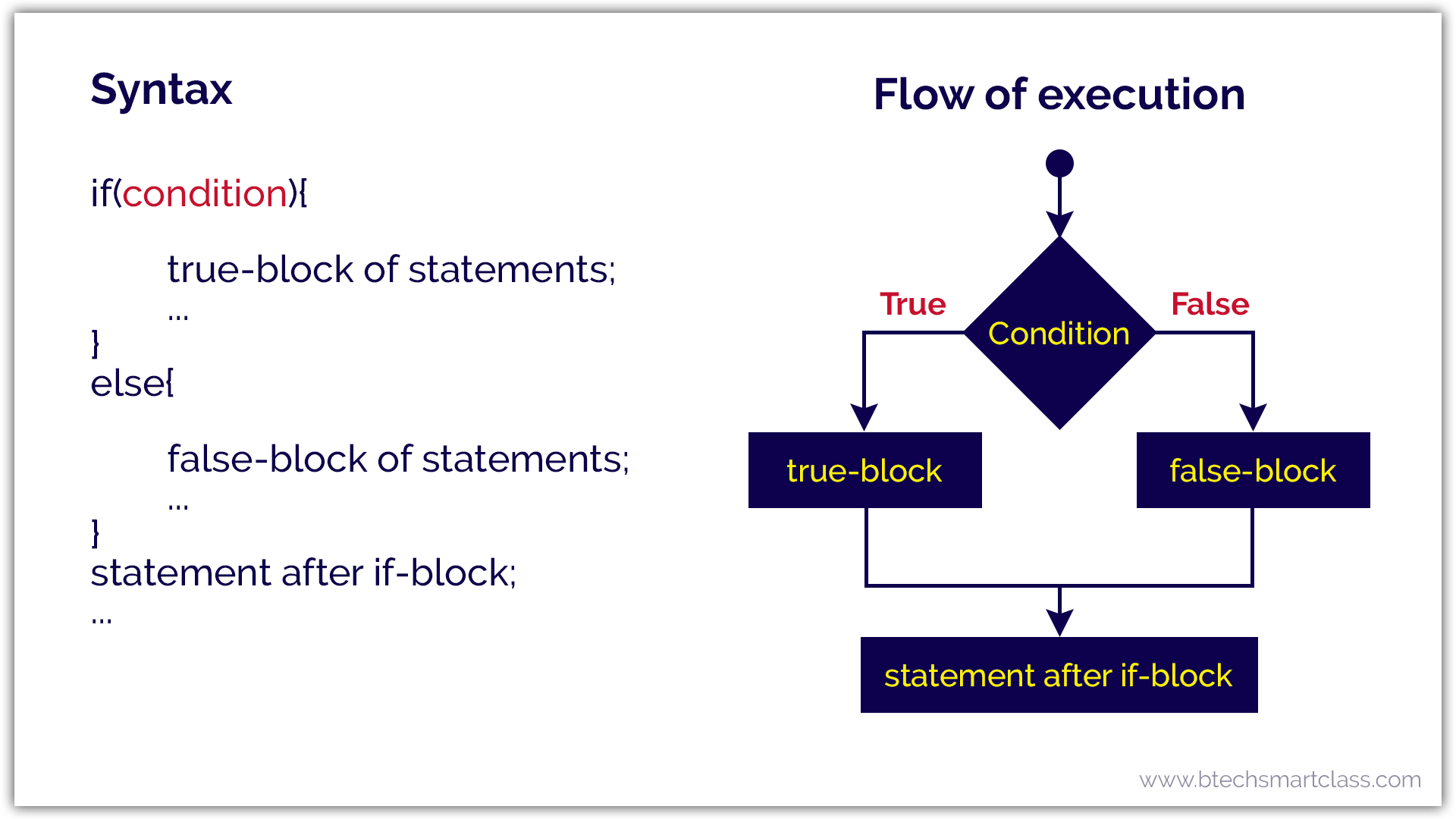Let's look at the following example java code.

Java Program
``````import java.util.Scanner;

public class IfElseStatementTest {

public static void main(String[] args) {

System.out.print("Enter any number: ");

if((num % 2) == 0) {
System.out.println("We are inside the true-block!");
System.out.println("Given number is EVEN number!!");
}
else {
System.out.println("We are inside the false-block!");
System.out.println("Given number is ODD number!!");
}

System.out.println("We are outside the if-block!!!");

}

}```
```

When we run this code, it produce the following output.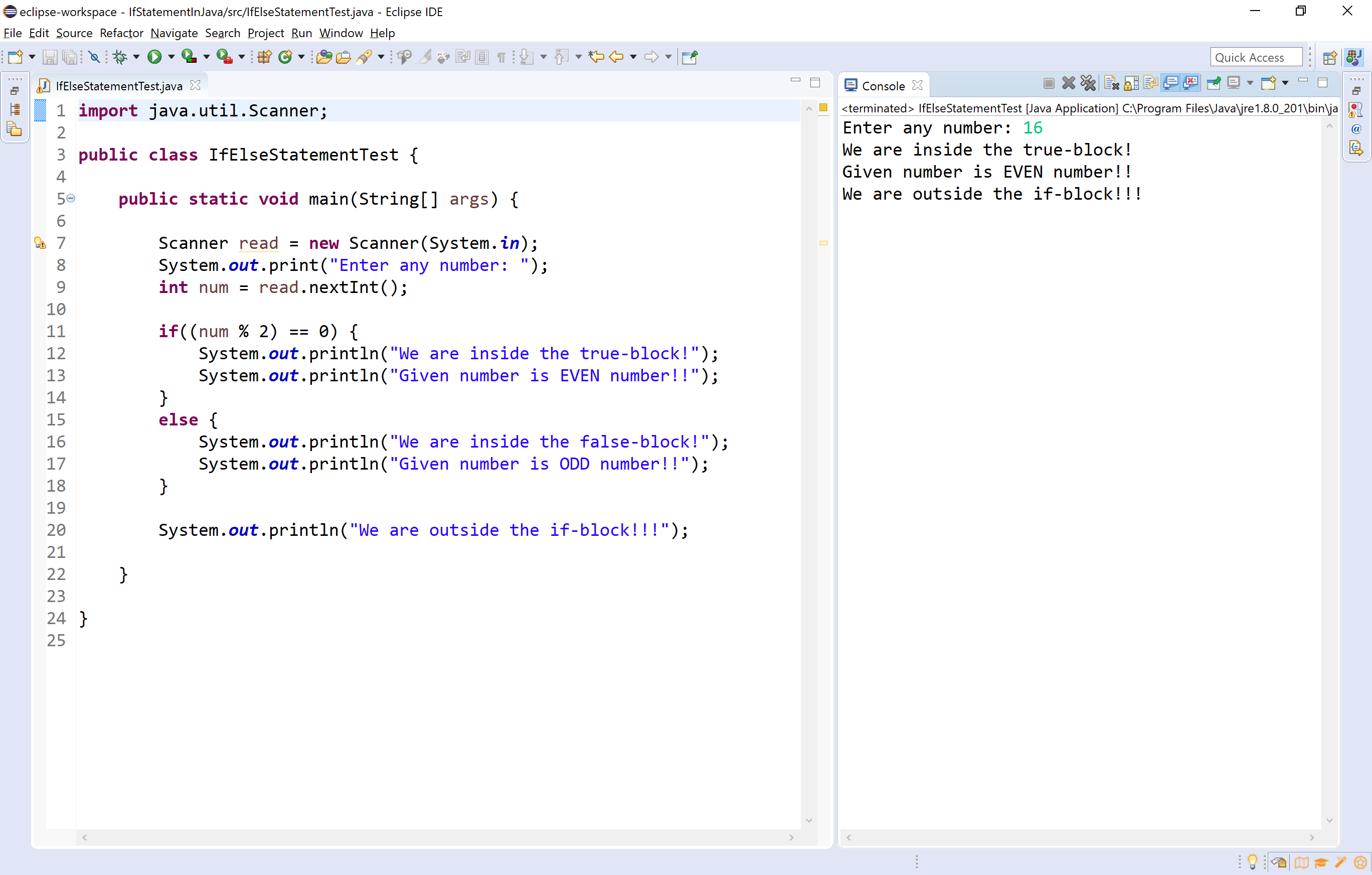## Nested if statement in java

Writing an if statement inside another if-statement is called nested if statement. The general syntax of the nested if-statement is as follows.

Syntax
``````if(condition_1){
if(condition_2){
inner if-block of statements;
...
}
...
}```
```

Let's look at the following example java code.

Java Program
``````import java.util.Scanner;

public class NestedIfStatementTest {

public static void main(String[] args) {

System.out.print("Enter any number: ");

if (num < 100) {
System.out.println("\nGiven number is below 100");
if (num % 2 == 0)
System.out.println("And it is EVEN");
else
System.out.println("And it is ODD");
} else
System.out.println("Given number is not below 100");

System.out.println("\nWe are outside the if-block!!!");

}

}```
```

When we run this code, it produce the following output.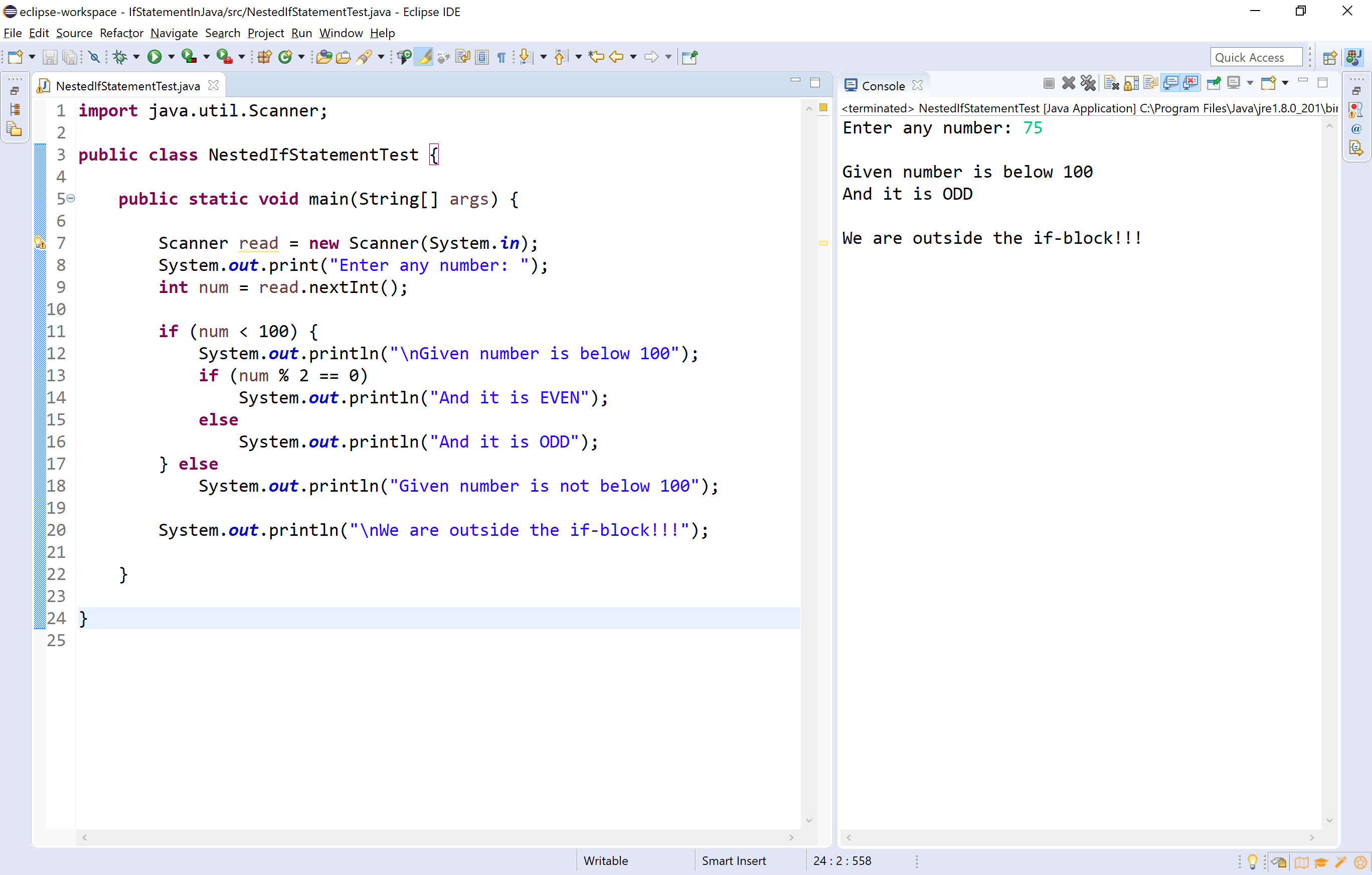## if-else if statement in java

Writing an if-statement inside else of an if statement is called if-else-if statement. The general syntax of the an if-else-if statement is as follows.

Syntax
``````if(condition_1){
condition_1 true-block;
...
}
else if(condition_2){
condition_2 true-block;
condition_1 false-block too;
...
}```
```

Let's look at the following example java code.

Java Program
``````import java.util.Scanner;

public class IfElseIfStatementTest {

public static void main(String[] args) {

int num1, num2, num3;
System.out.print("Enter any three numbers: ");

if( num1>=num2 && num1>=num3)
System.out.println("\nThe largest number is " + num1) ;

else if (num2>=num1 && num2>=num3)
System.out.println("\nThe largest number is " + num2) ;

else
System.out.println("\nThe largest number is " + num3) ;

System.out.println("\nWe are outside the if-block!!!");

}

}```
```

When we run this code, it produce the following output.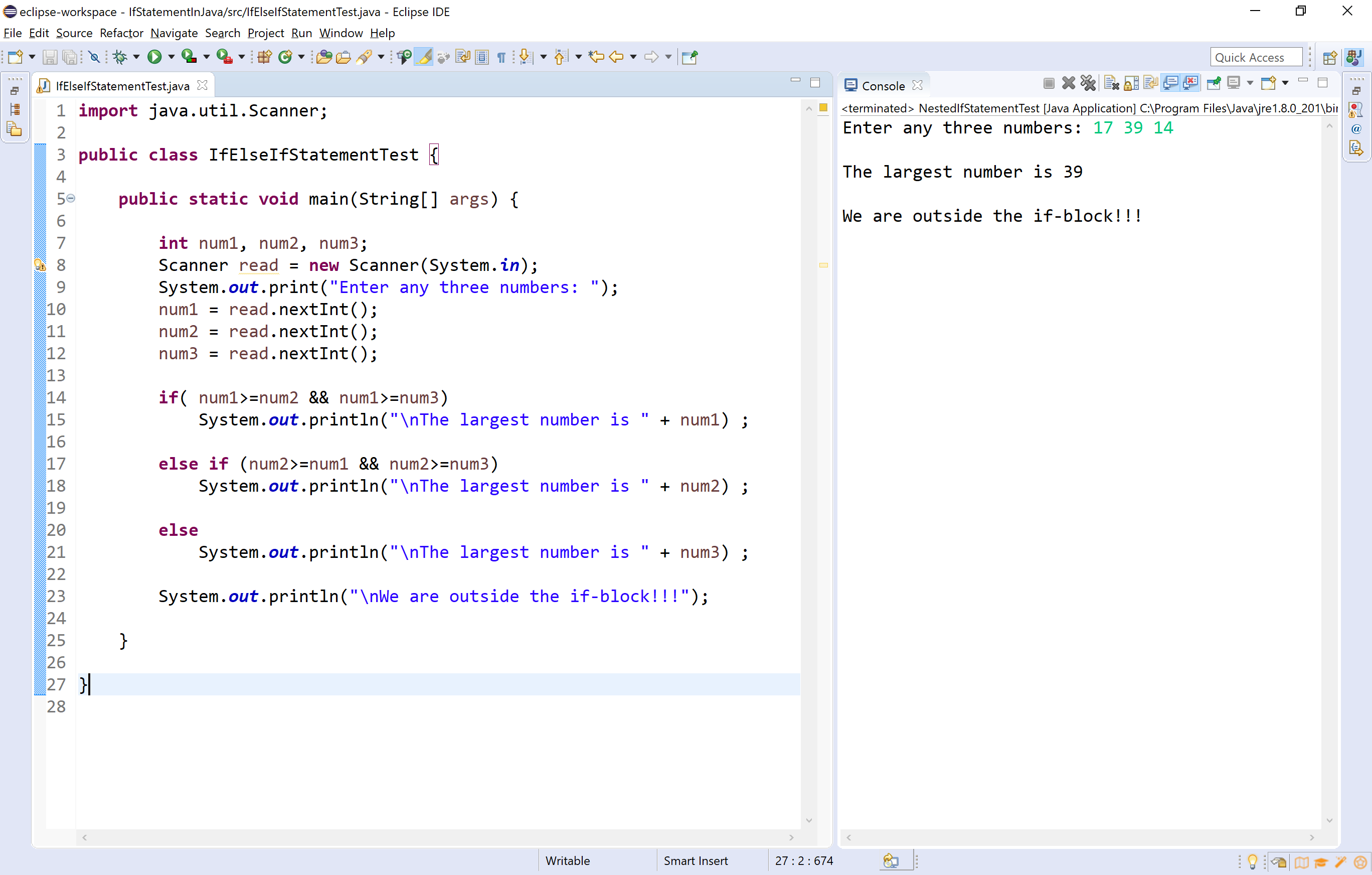## switch statement in java

Using the switch statement, one can select only one option from more number of options very easily. In the switch statement, we provide a value that is to be compared with a value associated with each option. Whenever the given value matches the value associated with an option, the execution starts from that option. In the switch statement, every option is defined as a case.

The switch statement has the following syntax and execution flow diagram.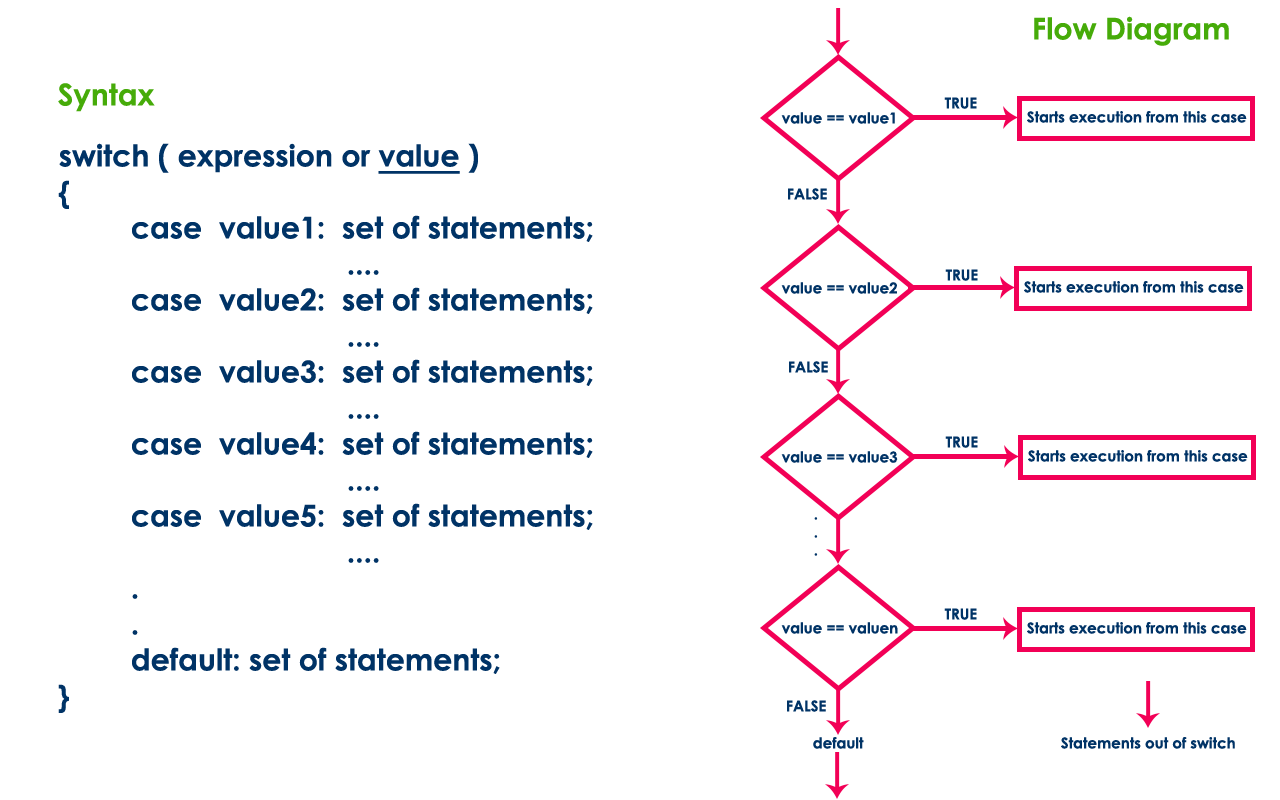Let's look at the following example java code.

Java Program
``````import java.util.Scanner;

public class SwitchStatementTest {

public static void main(String[] args) {

System.out.print("Press any digit: ");

switch( value )
{
case 0: System.out.println("ZERO") ; break ;
case 1: System.out.println("ONE") ; break ;
case 2: System.out.println("TWO") ; break ;
case 3: System.out.println("THREE") ; break ;
case 4: System.out.println("FOUR") ; break ;
case 5: System.out.println("FIVE") ; break ;
case 6: System.out.println("SIX") ; break ;
case 7: System.out.println("SEVEN") ; break ;
case 8: System.out.println("EIGHT") ; break ;
case 9: System.out.println("NINE") ; break ;
default: System.out.println("Not a Digit") ;
}

}

}```
```

When we run this code, it produce the following output.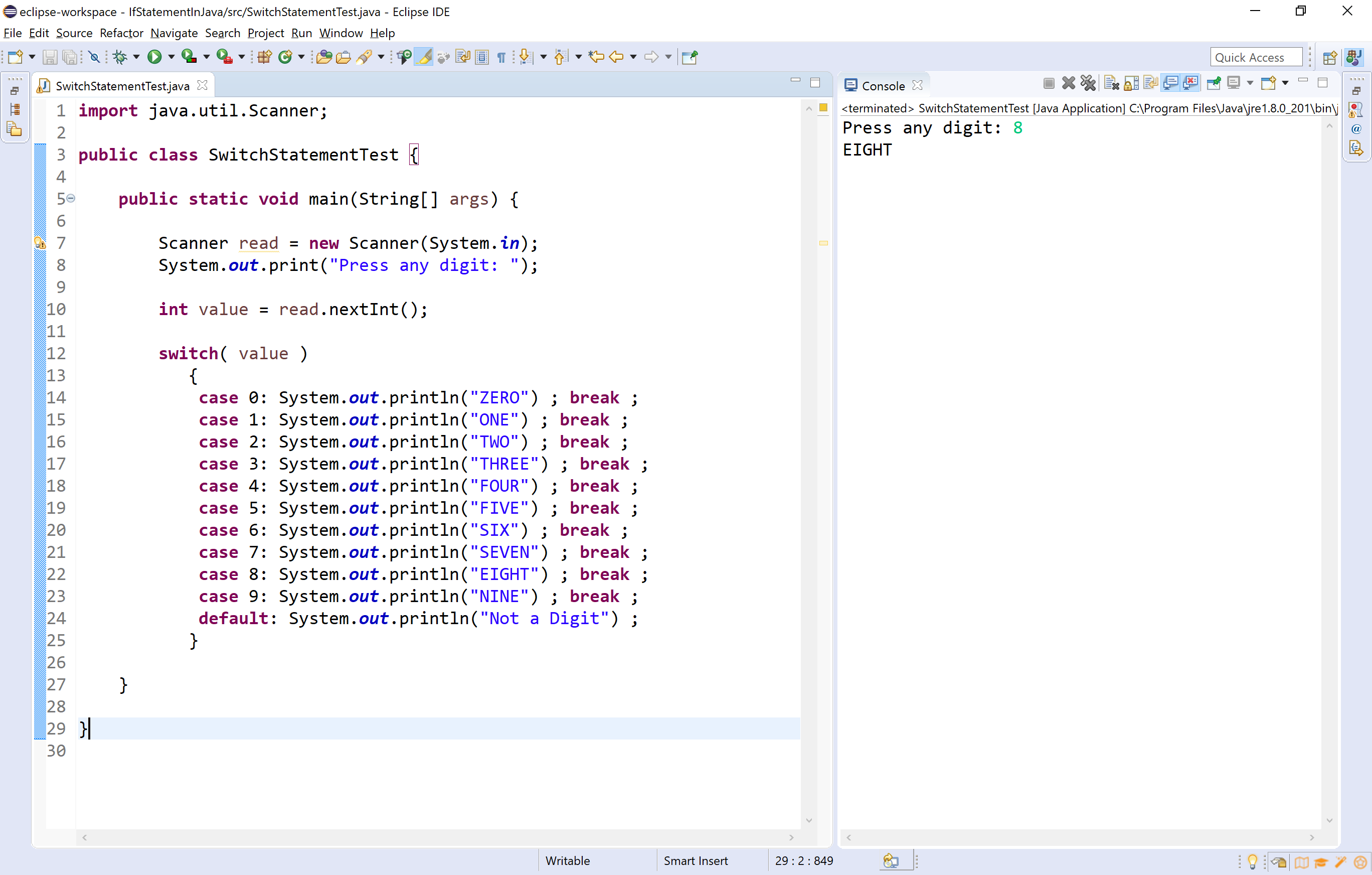🔔 In java, the case value of a switch statement can be a String value.